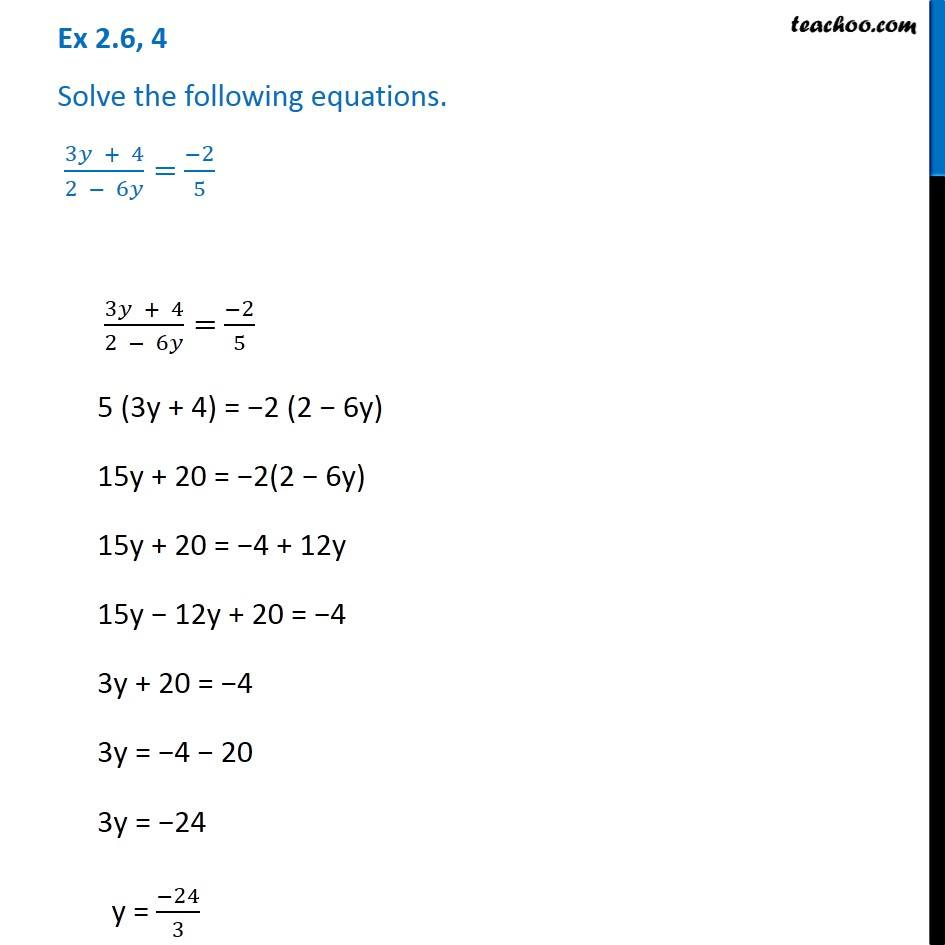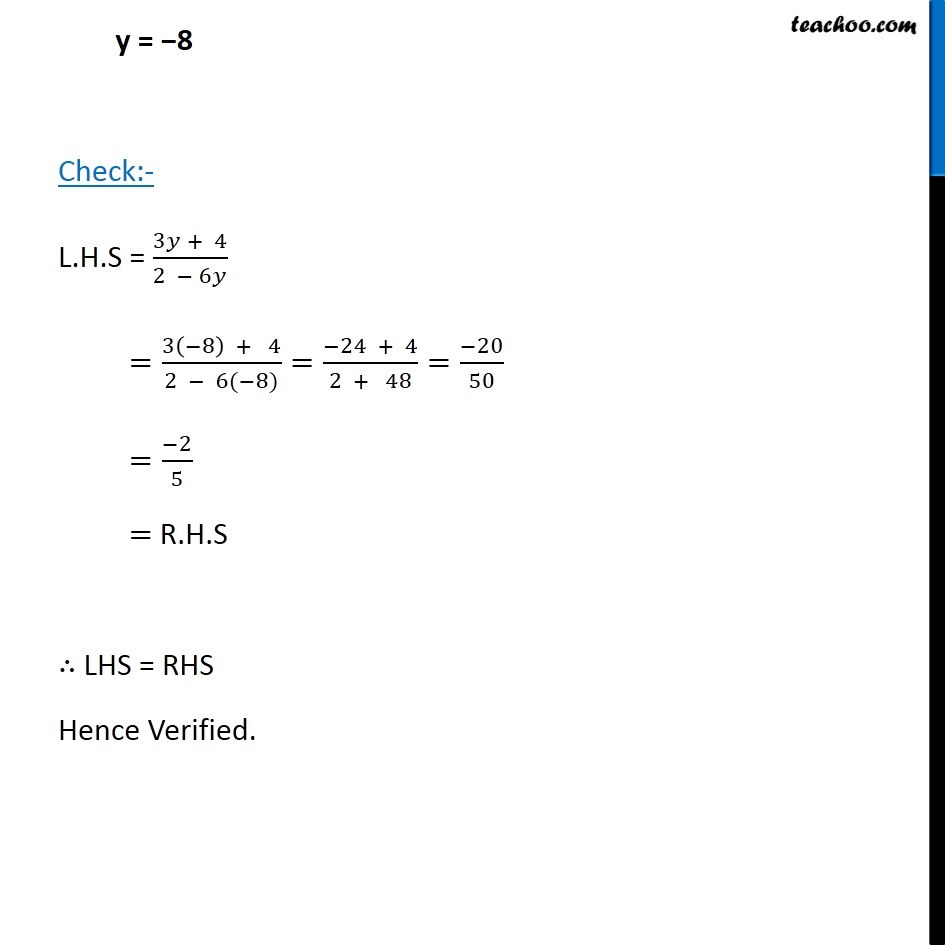Solving difficult equations - Word Problems

Chapter 2 Class 8 Linear Equations in One Variable
Serial order wiseLearn in your speed, with individual attention - Teachoo Maths 1-on-1 Class

### Transcript

Question 4 Solve the following equations. (3 + 4)/(2 6 )= ( 2)/5 (3 + 4)/(2 6 )= ( 2)/5 5 (3y + 4) = 2 (2 6y) 15y + 20 = 2(2 6y) 15y + 20 = 4 + 12y 15y 12y + 20 = 4 3y + 20 = 4 3y = 4 20 3y = 24 y = ( 24)/3 y = 8 Check:- L.H.S = (3 + 4)/(2 6 ) =(3( 8) + 4)/(2 6( 8))=( 24 + 4)/(2 + 48)=( 20)/50 =( 2)/5 = R.H.S LHS = RHS Hence Verified.